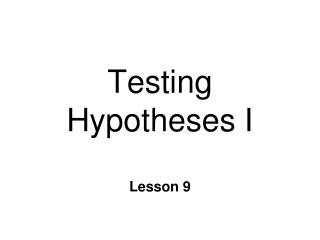DownloadDownload PresentationTesting Hypotheses I

# Testing Hypotheses I

Download Presentation## Testing Hypotheses I

- - - - - - - - - - - - - - - - - - - - - - - - - - - E N D - - - - - - - - - - - - - - - - - - - - - - - - - - -
##### Presentation Transcript

1. Testing Hypotheses I Lesson 9

2. Descriptive vs. Inferential Statistics • Descriptive • quantitative descriptions of characteristics • Inferential Statistics • Drawing conclusions about parameters ~

3. Hypothesis Testing • Hypothesis • testable assumption about a parameter • should conclusion be accepted? • final result a decision: YES or NO • qualitative not quantitative • General form of test statistic ~

4. Hypothesis Test: General Form

5. Evaluating Hypotheses Hypothesis: sample comes from this population

6. Two Hypotheses • Testable predictions • Alternative Hypothesis: H1 • also scientific or experimental hypothesis • there is a difference between groups • Or there is an effect • Reflects researcher’s prediction • Null Hypothesis: H0 • there is no difference between groups • Or there is no effect • This is hypothesis we test ~

7. Conclusions about Hypotheses • Cannot definitively “prove” or “disprove” • Logic of science built on “disproving” • easier than “proving” • State 2 mutually exclusive & exhaustive hypotheses • if one is true, other cannot be true • Testing H0 • Assuming H0 is true, what is probability we would obtain these data? ~

8. Hypothesis Test: Outcomes • Reject Ho • accept H1 as true • supported by data • statistical significance • difference greater than chance • Fail to reject • “Accepting” Ho • data are inconclusive ~

9. Hypotheses & Directionality • Directionality affects decision criterion • Direction of change of DV • Nondirectional hypothesis • Does reading to young children affect IQ scores? • Directional hypothesis • Does reading to young children increase IQ scores? ~

10. Nondirectional Hypotheses • 2-tailed test • Similar to confidence interval • Stated in terms of parameter • Hypotheses • H1 :m¹ 100 • Ho : m = 100 • Do not know what effect will be • can reject H0 if increase or decrease in IQ scores ~

11. Directional Hypotheses • 1- tailed test • predict that effect will be increase or decrease • Only predict one direction • Prediction of direction reflected in H1 • H1: m > 100 • Ho: m< 100 • Can only reject H0 if change is in same direction H1 predicts ~

12. Errors • “Accept” or reject Ho • only probability we made correct decision • also probability made wrong decision • Type I error (a) • incorrectly rejecting Ho • e.g., may think a new antidepressant is effective, when it is NOT ~

13. Errors • Type II error (b) • incorrectly “accepting” Ho • e.g., may think a new antidepressant is not effective, when it really is • Do not know if we make error • Don’t know true population parameters • *ALWAYS some probability we are wrong • P(killed by lightning)  1/1,000,000 • p = .000001 • P(win powerball jackpot)  1/100,000,000 ~

14. H0 is true H0 is false Reject H0 Errors Actual state of nature Type I Error Correct Decision Type II Error Accept H0 Correct

15. Definitions & Symbols • a • Level of significance • Probability of Type I error • 1 - a • Level of confidence • b • Probability of Type II error • 1 - b • Power ~

16. Steps in Hypothesis Test • State null & alternative hypotheses • Set criterion for rejecting H0 • Collect sample; compute sample statistic & test statistic • Interpret results is outcome statistically significant? ~

17. Example: Nondirectional Test • Experimental question: Does reading to young children affect IQ scores? • m = 100, s = 15, n = 25 • We will use z test • Same as computing z scores for ~

18. Step 1: State Hypotheses • H0: m = 100 • Reading to young children will not affect IQ scores. • H1: m 100 • Reading to young children will affect IQ scores. ~

19. 2. Set Criterion for Rejecting H0 • Determine critical value of test statistic • defines critical region(s) • Critical region • also called rejection region • area of distribution beyond critical value • in tails • If test statistic falls in critical region Reject H0 ~

20. 2. Set Criterion for Rejecting H0 • Level of Significance (a) • Specifies critical region • area in tail(s) • Defines low probability sample means • Most common: a = .05 • others: .01, .001 • Critical value of z • use z table for alevel ~

21. -2 -1 0 +1 +2 -1.96 +1.96 Critical Regions a = .05 zCV = + 1.96 f

22. 3. Collect data & compute statistics • Compute sample statistic • Observed value of test statistic • Need to calculate ~

23. 3. Collect sample & compute statistics n = 25 = assume : 105.5

24. -2 -1 0 +1 +2 -1.96 +1.96 Critical Regions a = .05 zCV = + 1.96 f

25. 4. Interpret Results • Is zobsin the critical region? • NO • we fail to reject H0 • These data suggest reading to young children does not affect IQ. • No “significant” difference • does not mean they are equal • data inconclusive ~